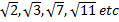Types of Numbers

# 1.Natural Numbers

Natural Numbers are counting Numbers. They are denoted by N. For example : N ={1,2,3…..}.
·         All natural numbers are positive
·         Zero is not a natural number. Therefore, 1 is the smallest natural number.

# 2.Whole Numbers

All natural numbers and zero form the set of whole numbers. Whole numbers are denoted by W. For example: W = {0,1,2,3…..}
·         Zero is the smallest whole number.
·         Whole numbers are also called as non-negative integers.

# 3.Integers

Whole numbers and negative numbers form the set of integers. They are denoted by I. For example : I ={….., -4, -3, -2, -1, 0 ,1, 2, 3, 4,….}
Integers are of two types.
(1)    Positive Integers : Natural numbers are called as positive integers. They are denoted by I+ . For Example : I+ ={1,2,3,4….}
(2)    Negative Integers : Negative of natural numbers are called as Negative Integers. They are denoted by I-. For example : I- = {-1,-2,-3,….}

# 4.Even Numbers

A counting number which is divisible by 2 is called an even number. For example : 2,4,6,8,10,12,…. Etc.
·         The unit’s place of every even number will be 0,2,4,6 or 8.

# 5.Odd Numbers

A counting Number which is not divisible by 2 is known as an Odd Number. For example: 1,3,5,7,9,11,13,15….etc.
·         The unit’s place of every odd number will be 1,3,5,7 or 9.

# 6.Prime numbers

A counting number which is called a prime number when it is exactly divisible by 1 and itself. For example: 2,3,5,7,11,13…etc.
·         2 is the only even number which is prime. It’s thus the smallest prime number as well.
·         A prime number is always greater than 1.
·         1 is not a prime number . Therefore, the lowest odd prime number is 3.
·         Every prime number greater than 3 can be represented by 6n+1 where n is an integer.

# 7.Composite Numbers

Composite numbers are non-prime natural numbers. They must have atleast one factor apart from 1 and itself. For example: 4,6,8,9, etc.
·         Composite numbers can be both odd and even.
·         2 is not a composite number.
·         1 is neither composite nor a prime number.

# 8.Co-Primes Numbers

Two natural numbers are said to be coprimes, if their HCF is 1. For example :(7,9), (15,16) etc.
·         Coprime numbers may or may not be prime.

# 9.Rational Numbers

A number that can be expressed as p/q is called a rational number , where p and q are integers and q is not equal to 0. For example : 3/5, 7/9, 13/15 etc

# 10.Irrational Numbers

The numbers that cannot be expressed in the form of p/q are called Irrational numbers, where p and q are integers and q is not equal to 0. For example -·# 11.Real Numbers

Real Numbers include rational and irrational numbers both. For example: 7/9,·         Real Numbers are denoted by R.